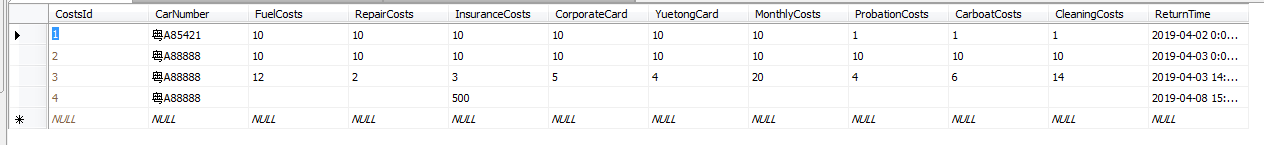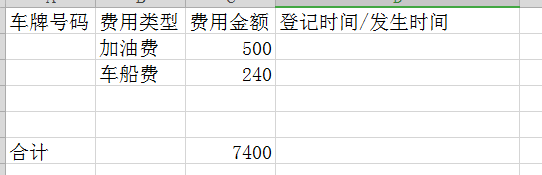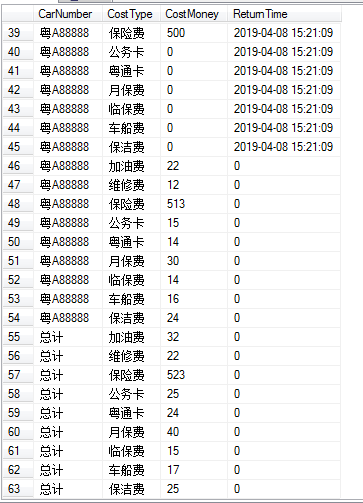•闪存
•博客
• 发言小组
• 投递新闻
• 提问博问
• 添加收藏
•文库

# 如何统计出费用？

0悬赏园豆：20 [待解决问题]select CarNumber,CostType,CostMoney,ReturnTime from
(select case when CarNumber is not null then CarNumber else '总计' end CarNumber,
isnull(convert(varchar(100),ReturnTime,120),0) as ReturnTime,
case when sum(cast(FuelCosts as int)) is not null then sum(cast(FuelCosts as int)) else null end 加油费,
case when sum(cast(RepairCosts as int)) is not null then sum(cast(RepairCosts as int)) else null end 维修费 ,
case when sum(cast(InsuranceCosts as int)) is not null then sum(cast(InsuranceCosts as int)) else null end 保险费 ,
case when sum(cast(CorporateCard as int)) is not null then sum(cast(CorporateCard as int))else null end 公务卡 ,
case when sum(cast(YuetongCard as int)) is not null then sum(cast(YuetongCard as int)) else null end 粤通卡,
case when sum(cast(MonthlyCosts as int)) is not null then sum(cast(MonthlyCosts as int)) else null end 月保费,
case when sum(cast(ProbationCosts as int)) is not null then sum(cast(ProbationCosts as int))else null end 临保费 ,
case when sum(cast(CarboatCosts as int)) is not null then sum(cast(CarboatCosts as int))else null end 车船费,
case when sum(cast(CleaningCosts as int)) is not null then sum(cast(CleaningCosts as int)) else null end 保洁费
FROM CarCosts group by CarNumber,ReturnTime with rollup ) as a
unpivot(CostMoney for CostType in (加油费,维修费,保险费,公务卡,粤通卡,月保费,临保费,车船费,保洁费)) as b0

您需要登录以后才能回答，未注册用户请先注册